imcolordiff

Color difference based on CIE94 or CIE2000 standard

Description

example

dE = imcolordiff(I1,I2) calculates the color difference between two RGB images or color maps using the CIE94 standard.

example

dE = imcolordiff(I1,I2,Name,Value) specifies additional aspects of the computation, such as the input color space and the CIE standard, using one or more name-value pair arguments.

Examples

collapse all

Read a color image into the workspace.

imshow(I1)Alter the local color contrast in the image.

I2 = localcontrast(I1);
imshow(I2)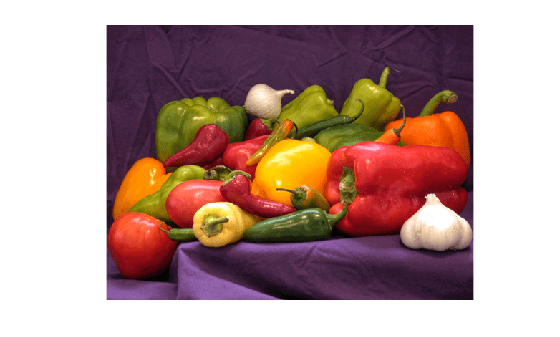Calculate the color difference of the images using the default color standard, CIE94.

dE = imcolordiff(I1,I2);

Display the color difference as an image. Scale the display range to use the full range of pixel values in dE.

imshow(dE,[])Read and display an image of tissue stained with hemotoxylin and eosin (H&E).

imshow(he)Convert the image to the L*a*b* color space.

lab = rgb2lab(he);

Make a copy of the image, then increase the signal of the a* channel. Red tones in the image become more saturated while the image overall brightness and the blue tones are unchanged.

lab2 = lab;
scaleFactor = 1.1;
lab2(:,:,2) = scaleFactor*lab(:,:,2);

Calculate the color difference of the original and enhanced image in the L*a*b* color space.

dE = imcolordiff(lab,lab2,'isInputLab',true);

Display the color difference as an image. Scale the display range to match the range of pixel values in dE. Bright regions indicate the greatest color difference and correspond with the pink regions of tissue.

imshow(dE,[])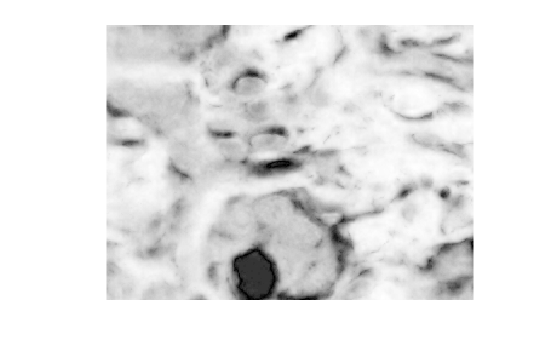Specify two RGB color values.

pureRed = uint8([255,0,0]);
darkRed = uint8([255,10,50]);

Calculate the color difference of the colors using the CIEDE2000 standard.

dE = imcolordiff(pureRed,darkRed,"Standard","CIEDE2000")
dE = single
7.4449

Read and display an RGB image of fabric.

imshow(fabric)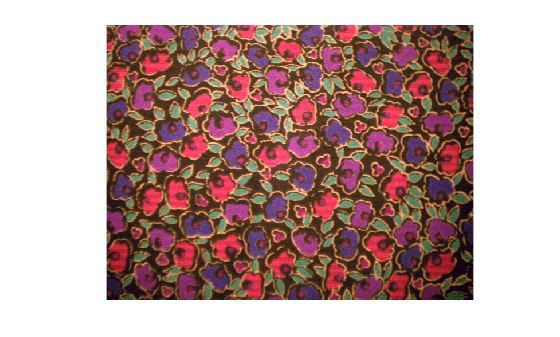Simulate a second image of fabric by altering the local color contrast in the image.

fabric2 = localcontrast(fabric);
imshow(fabric2)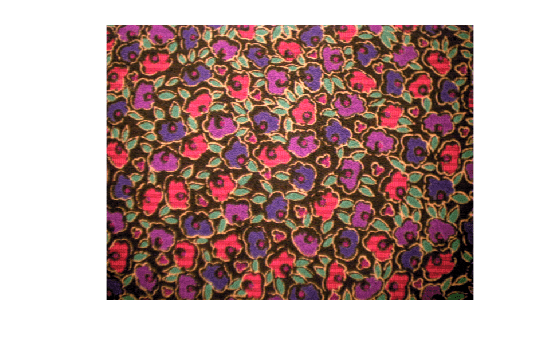Calculate the color difference of the two images using the CIEDE2000 standard. Specify a luminance coefficient and K1 and K2 weighting factors appropriate for textiles.

dE = imcolordiff(fabric,fabric2,'Standard','CIEDE2000', ...
'kL',2,'K1',0.048,'K2',0.014);

Display the color difference. Scale the display range to the full range of pixel values in dE.

imshow(dE,[])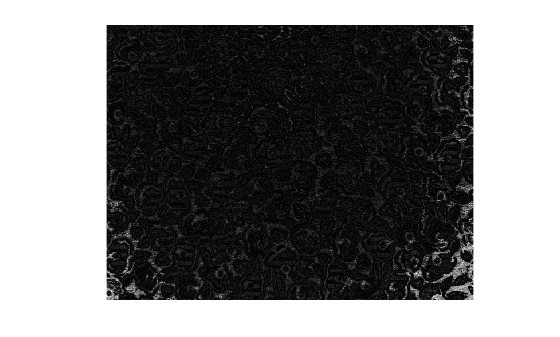Input Arguments

collapse all

First set of color data, specified as an m-by-n-by-3 numeric array representing an image or a c-by-3 numeric matrix representing a set of c colors. I1 and I2 must be the same size with values in the same color space.

By default, the imcolordiff function interprets the color data as RGB color values. To calculate the color difference in the L*a*b* color space, specify the 'isInputLab' argument as true. L*a*b* color values can be of data type single or double only.

Data Types: single | double | uint8 | uint16

Second set of color data, specified as an m-by-n-by-3 numeric array representing an image or a c-by-3 numeric matrix representing a set of c colors. I1 and I2 must be the same size with values in the same color space.

By default, imcolordiff interprets the color data as RGB color values. To calculate the difference of colors in the L*a*b* color space, specify the 'isInputLab' argument as true. L*a*b* color values can be of data type single or double only.

Data Types: single | double | uint8 | uint16

Name-Value Arguments

Specify optional comma-separated pairs of Name,Value arguments. Name is the argument name and Value is the corresponding value. Name must appear inside quotes. You can specify several name and value pair arguments in any order as Name1,Value1,...,NameN,ValueN.

Example: 'Standard',"CIEDE2000" calculates the color difference between two RGB images using the CIEDE2000 standard.

CIE standard used to compute the color difference value, specified as the comma-separated pair consisting of 'Standard' and one of these values:

ValueDescription
"CIE94"The CIE94 standard. This standard improves the perceptual non-uniformities of the CIE76 standard implemented in the deltaE function.
"CIEDE2000"The CIEDE2000 standard. This standard further improves the perceptual uniformity through five additional corrections: a hue rotation term, compensation for neutral colors, and compensation for lightness, chroma, and hue.

Data Types: char | string

Color values are in the L*a*b* color space, specified as the comma-separated pair consisting of 'isInputLab' and a numeric or logical 0 (false) or 1 (true).

Luminance coefficient, specified as the comma-separated pair consisting of 'kL' and a numeric scalar. The luminance coefficient is typically 1 for applications in graphic arts and 2 for applications in textiles.

K1 weighting factor, specified as the comma-separated pair consisting of 'K1' and a numeric scalar. The K1 weighting factor is typically 0.045 for applications in graphic arts and 0.048 for applications in textiles.

K2 weighting factor, specified as the comma-separated pair consisting of 'K2' and a numeric scalar. The K2 weighting factor is typically 0.015 for applications in graphic arts and 0.014 for applications in textiles.

Output Arguments

collapse all

Color difference (delta E), returned as one of the following.

• An m-by-n matrix when the input color data I1 and I2 represent images

• A c-element column vector when I1 and I2 represent a set of c colors

If I1 or I2 is of data type double, then dE is of data type double. Otherwise, dE is of data type single.

Data Types: single | double

Tips

• To calculate color differences following the CIE76 standard, use the deltaE function. This function is faster than the imcolordiff function, but less precise.

 Sharma, Gaurav, Wencheng Wu, and Edul N. Dalal, "The CIEDE2000 Color-Difference Formula: Implementation Notes, Supplementary Test Data, and Mathematical Observations". Color Research and Application 30, no. 1 (February 2005): 21–30. https://doi.org/10.1002/col.20070.